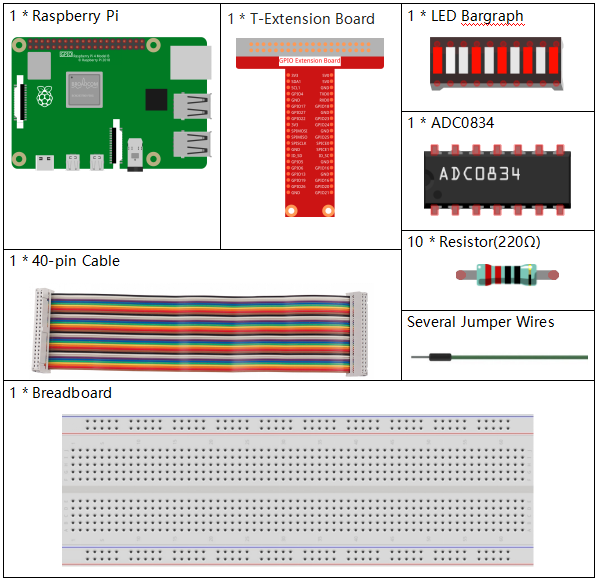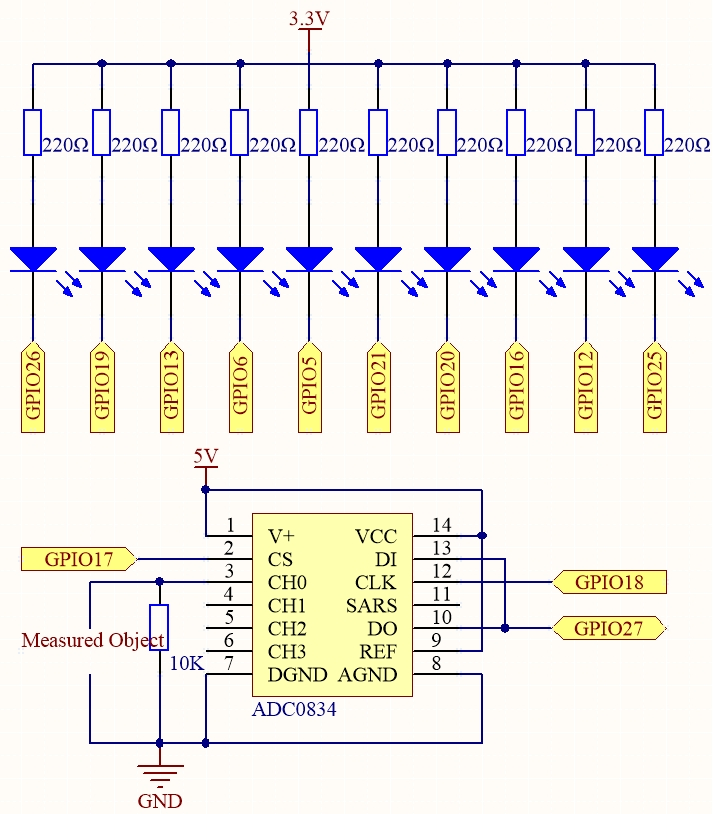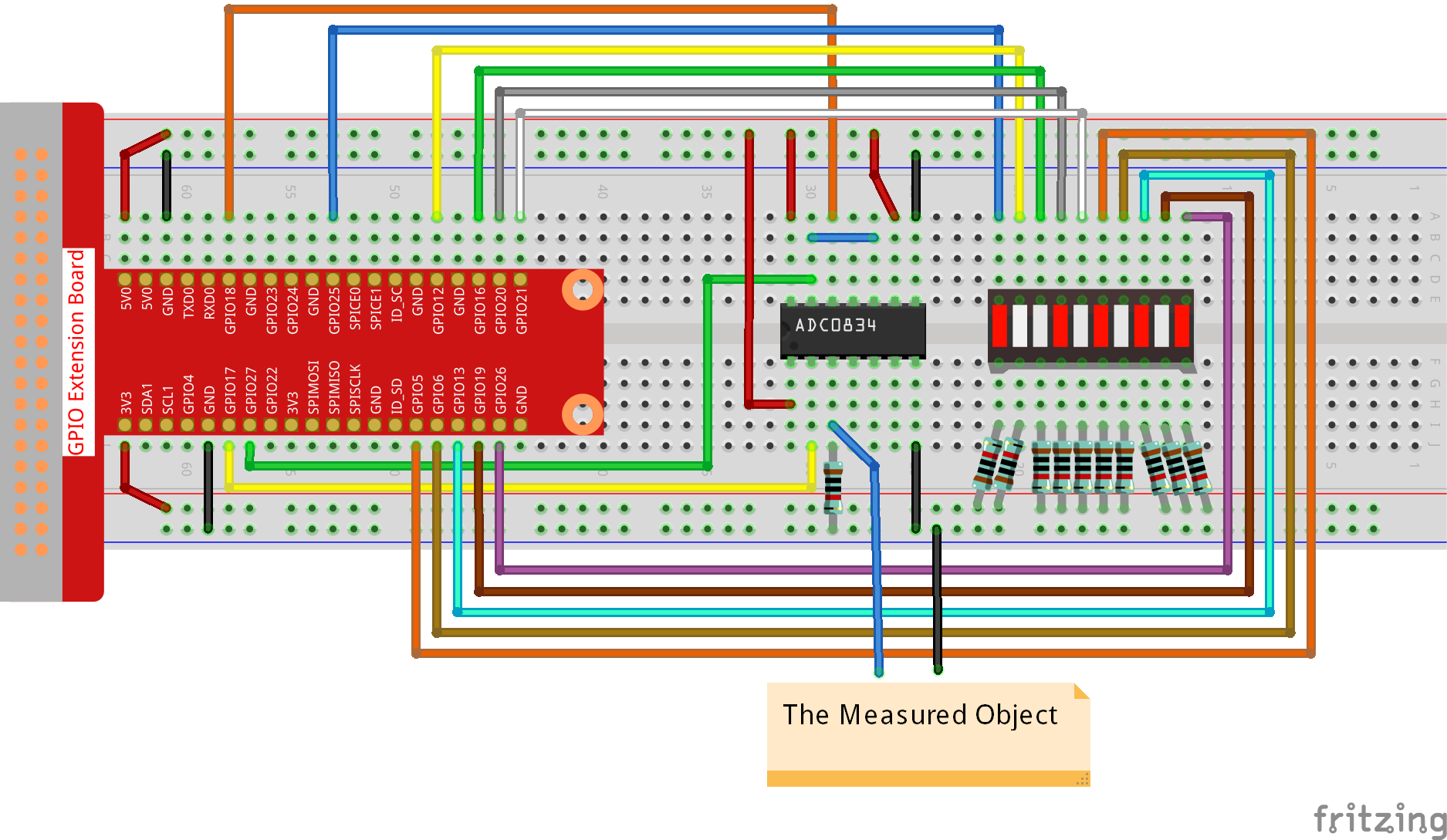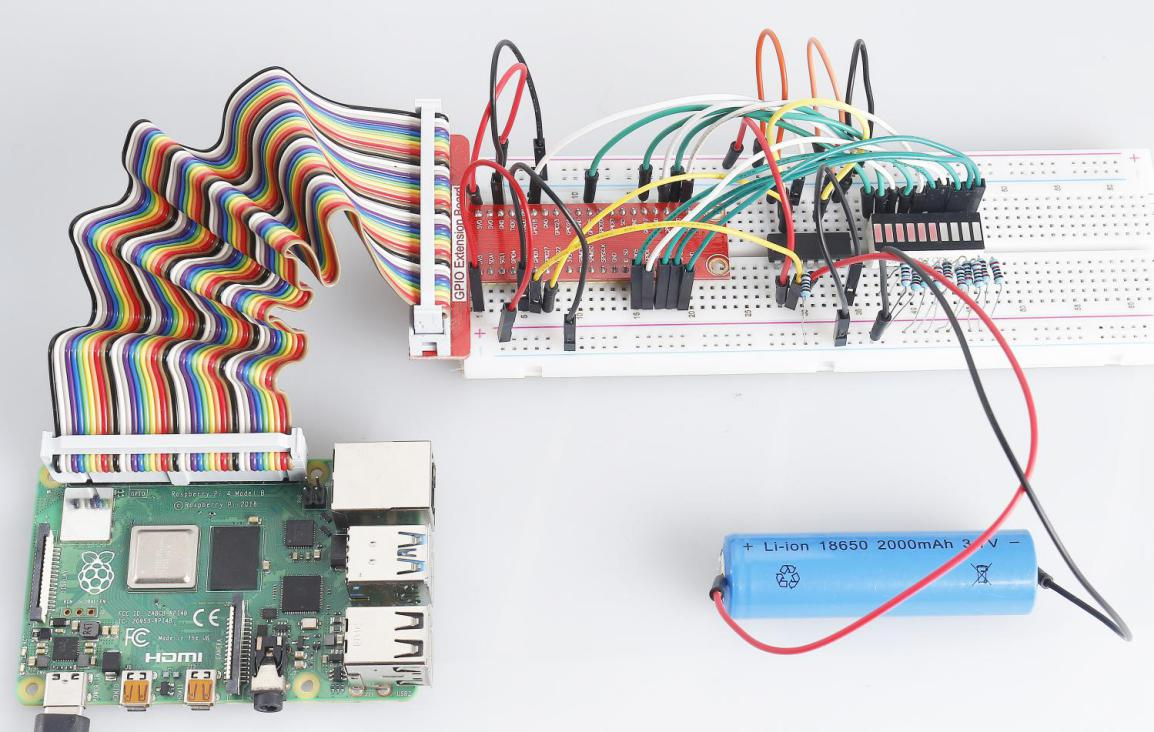# 3.1.5 Battery Indicator¶

## Introduction¶

In this course, we will make a battery indicator device that can visually display the battery level on the LED Bargraph.

## Components¶## Schematic Diagram¶

 T-Board Name physical wiringPi BCM GPIO17 Pin 11 0 17 GPIO18 Pin 12 1 18 GPIO27 Pin 13 2 27 GPIO25 Pin 22 6 25 GPIO12 Pin 32 26 12 GPIO16 Pin 36 27 16 GPIO20 Pin 38 28 20 GPIO21 Pin 40 29 21 GPIO5 Pin 29 21 5 GPIO6 Pin 31 22 6 GPIO13 Pin 33 23 13 GPIO19 Pin 35 24 19 GPIO26 Pin 37 25 26## Experimental Procedures¶

Step 1: Build the circuit.### For C Language Users¶

Step 2: Go to the folder of the code.

```cd /home/pi/davinci-kit-for-raspberry-pi/c/3.1.5/
```

Step 3: Compile the code.

```gcc 3.1.5_BatteryIndicator.c -lwiringPi
```

Step 4: Run the executable file.

```sudo ./a.out
```

After the program runs, give the 3rd pin of ADC0834 and the GND a lead-out wire separately and then lead them to the two poles of a battery separately. You can see the corresponding LED on the LED Bargraph is lit up to display the power level (measuring range: 0-5V).

Note

If it does not work after running, or there is an error prompt: “wiringPi.h: No such file or directory”, please refer to C code is not working?.

Code Explanation

```void LedBarGraph(int value){
for(int i=0;i<10;i++){
digitalWrite(pins[i],HIGH);
}
for(int i=0;i<value;i++){
digitalWrite(pins[i],LOW);
}
}
```

This function works for controlling the turning on or off of the 10 LEDs on the LED Bargraph. We give these 10 LEDs high levels to let they are off at first, then decide how many LEDs are lit up by changing the received analog value.

```int main(void)
{
uchar analogVal;
if(wiringPiSetup() == -1){ //when initialize wiring failed,print messageto screen
printf("setup wiringPi failed !");
return 1;
}
for(int i=0;i<10;i++){       //make led pins' mode is output
pinMode(pins[i], OUTPUT);
digitalWrite(pins[i],HIGH);
}
while(1){
LedBarGraph(analogVal/25);
delay(100);
}
return 0;
}
```

analogVal produces values (0-255) with varying voltage values (0-5V), ex., if a 3V is detected on a battery, the corresponding value 152 is displayed on the voltmeter.

The 10 LEDs on the LED Bargraph are used to display the analogVal readings. 255/10=25, so every 25 the analog value increases, one more LED turns on, ex., if “analogVal=150 (about 3V), there are 6 LEDs turning on.”

### For Python Language Users¶

Step 2: Go to the folder of the code.

```cd /home/pi/davinci-kit-for-raspberry-pi/python/
```

Step 3: Run the executable file.

```sudo python3 3.1.5_BatteryIndicator.py
```

After the program runs, give the 3rd pin of ADC0834 and the GND a lead-out wire separately and then lead them to the two poles of a battery separately. You can see the corresponding LED on the LED Bargraph is lit up to display the power level (measuring range: 0-5V).

Code

Note

You can Modify/Reset/Copy/Run/Stop the code below. But before that, you need to go to source code path like `davinci-kit-for-raspberry-pi/python`.

```import RPi.GPIO as GPIO
import time

ledPins = [25, 12, 16, 20, 21, 5, 6, 13, 19, 26]

def setup():
GPIO.setmode(GPIO.BCM)
for i in ledPins:
GPIO.setup(i, GPIO.OUT)
GPIO.output(i, GPIO.HIGH)

def LedBarGraph(value):
for i in ledPins:
GPIO.output(i,GPIO.HIGH)
for i in range(value):
GPIO.output(ledPins[i],GPIO.LOW)

def destroy():
GPIO.cleanup()

def loop():
while True:
LedBarGraph(int(analogVal/25))

if __name__ == '__main__':
setup()
try:
loop()
except KeyboardInterrupt: # When 'Ctrl+C' is pressed, the program destroy() will be executed.
destroy()
```

Code Explanation

```def LedBarGraph(value):
for i in ledPins:
GPIO.output(i,GPIO.HIGH)
for i in range(value):
GPIO.output(ledPins[i],GPIO.LOW)
```

This function works for controlling the turning on or off of the 10 LEDs on the LED Bargraph. We give these 10 LEDs high levels to let they are off at first, then decide how many LEDs are lit up by changing the received analog value.

```def loop():
while True: